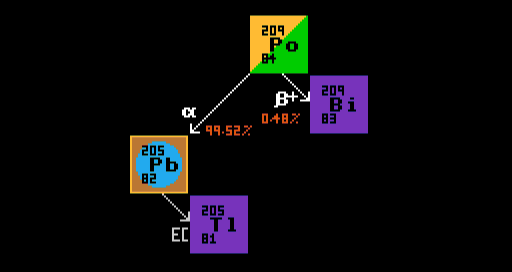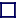### MULTI-MODAL DECAYS

MULTI-MODAL DECAYS, BRANCHING RATIO AND DECAY CONSTANT
When an nuclide can decay by more than one pathway, it is said to exhibit multi-modal decay. But the halflife of a nuclide takes account only of the rate of change of the parent nuclide, and not of the rate of decay along different pathways possible in multi-modal decay. For multi-modal decays, the branching ratio (or branching fraction) awards percentage probabilities to each modal pathway. The decay constant (or transition rate) takes into account the 'partial halflives' of each different route, and is related to the halflife and branching ratio by the following equation:

 Decay Constant =Branching Ratio x (0.5)(1/Halflife) Decay Constant = 1 / Mean Lifetime Mean Lifetime = Halflife / LOGe(2)
In the above diagram, Rhenium-186 (the central isotope) exhibits a bi-modal decay; when it decays, it has a 93% probability of taking the beta decay route to become osmium-186 (itself radioactive) and an 7% probability of taking the electron capture pathway to become stable tungsten-186. The branching ratio is therefore 93% Beta, 7% EC. The (overall) halflife is 88.9 hours, corresponding to an overall decay constant of 0.78% per hour. The decay constant for the beta pathway is 93% of this overall value (0.73%/hour) and that for the electron capture pathway 7% of the overall value (0.05%/hour).

Overall Decay constant = Decay constant(branch 1) + Decay constant(branch 2)

A second example below shows the multi-modal decay of polonium-209, which decays by either alpha decay into lead-205, or by inverse beta decay into bismuth-209. The branching ratio for this is 99.52% alpha decay; 0.48% inverse beta decay. Therefore, after an infinite amount of time, 0.48% of the number of atoms of polonium-209 end up as atoms of the stable bismuth-209, whereas 99.52% ends up as atoms, not of lead-205, which is itself electron-capture radioactive, but of thallium-205, which is lead-205's stable daughter product. Observe that the same number of alpha particles as atoms of lead-205 are produced. Similarly, as many positrons are produced as are atoms of bismuth-209. [Note that branching ratios do not relate to the percentage weights, but rather to the percentage atoms relative to the number of atoms of the parent isotope, polonium-209 in this case].Multi-modal decay of polonium-209